Visitors Online: 77 | Tuesday 22nd October 2019

CBSE Guess > Papers > Question Papers > Class XII > 2006 > Mathematics > Delhi Set - II.

Mathematics — 2006 (Set II — Delhi)

SECFION - A

Q. 4.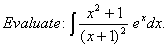Q. 6. Solve the following differential equation:Q. 8.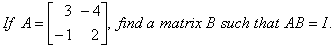Q. 11.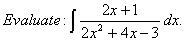Q. 13.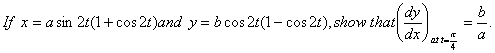Q. 18. Using matrices, solve the following system of equations:SECTION - B

Q. 22. If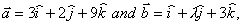find the value ofis perpendicular to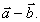Q. 23. Find the equation of the plane passing through the points (0, —1, —1), (4, 5, 1) and (3, 9, 4).

Or

Find the equation of the sphere on the join of points A and B with position vectors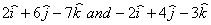respectively as diameter. Find the centre and radius of sphere also.

Q. 26. The vector equations of two lines are:SECTION - C

Q. 19. Find the face value of a bill discounted at 5% per annum 146 days earlier than the legal date of maturity when the banker’s gain is Rs. 5.

Q. 23. Find the amount of an annuity of Rs. 500 payable at the end of every month for 3 years if the money is worth 15% per annum, compounded monthly. [Use (1.0125)36= 1.5391]

 Mathematics 2006 Question Papers Class XII Delhi Outside Delhi Compartment Delhi Compartment Outside Delhi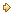Set ISet ISet ISet ISet IISet IISet IISet IISet IIISet III

 CBSE 2006 Question Papers Class XII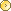EnglishSociologyFunctional EnglishPsychologyMathematicsPhilosopyPhysicsComputer ScienceChemistryEntrepreneurshipBiologyInformatics PracticesGeographyMultimedia & Web TechnologyEconomicsBiotechnologyBusiness StudiesPhysical EducationAccountancyFine ArtsPolitical ScienceHistoryAgriculture